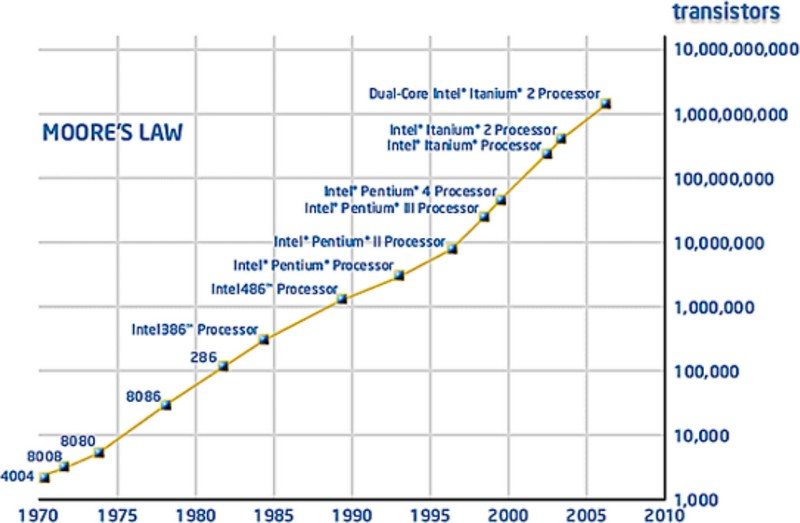# Fixed Point Number - Precision

Precision is the maximum number of significant digits (on either side of the radix point) in the fixed point notation

If a value exceeds the precision, then an application such as a database would return an error.

## Example

### Single number

• The number 00123456.78 has a precision of 8
• 123.45 has a precision of 5
• 1.2345 has also a precision of 5

### Decimal and precision

 Precision Rounded to significant figures Rounded to decimal places 6 12.3450 12.345000 5 12.345 12.34500 4 12.35 12.3450 3 12.3 12.345 2 12 12.35 1 10 12.3 0 N/A 12

Recommended PagesComputer - Floating-point (Representation and usage)

Computer representations of floating point numbers typically use a form of rounding to significant figures, but with binary numbers. The number of correct significant figures is closely related to the...Computer Architecture - Arithmetic logic unit (ALU)

The ALU is a component of a CPU. It is a device that executes all arithmetic and logical operations. In most arithmetic logic unit (ALU) hardware, we find a fixed-precision arithmetic found , which...Number - (Numeral system|System of numeration|Representation of number)

A numeral system (or system of numeration) is a mathematical notation system for expressing numbers using digits (or other symbols). The numeral system gives the context that allows the (digits|symbols)...Number - Fixed-point numbers (exact numbers)

A fixed-point number represents a limited-precision rational number that may have a fractional part. These numbers are stored internally in a scaled-integer form, typically in binary but sometimes in...Number - Significant (Figures|Digits|Bits)

A significant digit is a digit that can't be removed from the number without modifying its value. The most significant digit is the left-most nonzero digit The least significant digit is the...SQL - Decimal(p,s) or Numeric(p,s)

DECIMAL and NUMERIC are sql datatype used to represent a fixed point notation (exact number) numeric is generally functionally identical to decimal. With a DECIMAL(5,2): the number has: a precision...